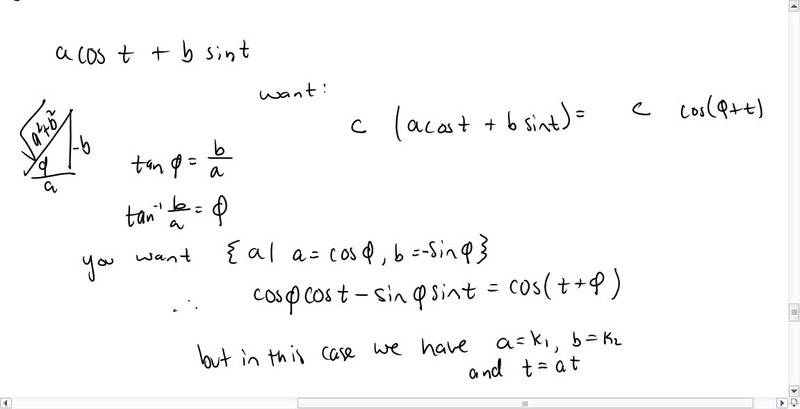Where does the phi come from in the charge of a capacitor in an LC circuit?

I'm reading University Physics 13e by Young and Freedman and we're given this equation:

-L(di/dt)-q/C=0

So we know that i, current, is, i=dq/dt. So the first equation can be read, after some simplification, as

q''+(1/LC)q=0

where q prime means the derivative of q (charge) with respect to time.

This is a homogeneous system so I figure solve it like anything else. So

s^2 = -(1/LC)
=>
s=i*(1/LC)^1/2..... the i here is imaginary

let: a=(1/LC)^1/2

thus q=k1*cos(at) + k2*sin(at)

Yet in the formula in the book we end up with the formula:
q=Q*cos(at + phi)

with no sine...

Any help?